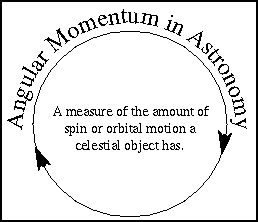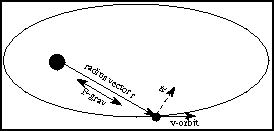# Appendix A

This appendix was copied with permission from Nick Strobel’s Astronomy Notes. Go to his site at www.astronomynotes.com for the updated and corrected version.

# Angular Momentum

I define angular momentum and give several examples of angular momentum in astronomy: Kepler’s second law of orbital motion, Earth-Moon system, rapidly spinning neutron stars, accretion disk in a binary system, and a collapsing galactic cloud.## Definition

To describe how things move we often use the basic quantities of length, mass, and time. Quantities such as velocity, acceleration, force and energy are very powerful ones that help us understand how an object’s position will change over time and how it will interact with other things in the universe. Momentum and its cousin angular momentum are other very powerful quantities.

Ordinary momentum is a measure of an object’s tendency to move at constant speed along a straight path. Momentum depends on speed and mass. A train moving at 20 mph has more momentum than a bicyclist moving at the same speed. A car colliding at 5 mph does not cause as much damage as that same car colliding at 60 mph. For things moving in straight lines momentum is simply mass × speed. In astronomy most things move in curved paths so we generalize the idea of momentum and have angular momentum. Angular momentum measures an object’s tendency to continue to spin. An “object” can be either a single body or two or more bodies acting together as a single group.

#### angular momentum = mass × velocity × distance (from point object is spinning or orbiting around)

Very often in astronomy, the object (or group of objects) we’re observing has no outside forces acting on it in a way to produce torques that would disturb the angular motion of the object (or group of objects). A torque is simply a force acting along a line that is off the object’s spin axis. In these cases, we have conservation of angular momentum.

#### conservation of angular momentum—the total amount of angular momentum does not change with time no matter how the objects interact with one another.

A planet’s velocity and distance from the Sun will change but the combination of speed×distance will not change unless another planet or star passes close by and provides an extra gravity force.

## Applications

### 1) Kepler’s Second Law of orbital motion

The area swept out by a line connecting an orbiting object and the central point is the same for any two equal periods of times. That line is called a radius vector in the following discussion.

The rate of change of the swept-out area does NOT change with time. The line along which gravity acts is parallel to the radius vector. This means that there are no torques disturbing the angular motion and, therefore, angular momentum is conserved. The part of the orbital velocity (v-orbit) perpendicular (at a right angle) to the radius vector (r) is vt. The rate of change of the swept-out area = r × vt / 2.

To calculate the orbital angular momentum use vt for the velocity. So, the angular momentum = mass × vt × r = mass × 2 × (rate of change of area). That value does not change over time. So if r decreases, v-orbit (and vt) must increase! If r increases, v-orbit (and vt) must decrease. This is just what Kepler observed for the planets!### 2) Earth-Moon system

The total angular momentum = spin angular momentum + orbital angular momentum. The total angular momentum is CONSTANT. To find the spin angular momentum, subdivide the object into small pieces of mass and find the angular momentum for each of the small pieces. Then add up the angular momentum for all of the pieces. The Earth’s spin speed is decreasing so its spin angular momentum is DEcreasing. Therefore, the Moon’s orbital angular momentum must compensate by INcreasing. It does this by increasing the Earth-Moon distance.### 3) Rapidly spinning neutron stars

Originally, a big star has a core 10,000’s to 100,000’s kilometers in radius (the whole star is even bigger!). Here the radius is used instead of the diameter, because what is important is how far each piece of the core is from the spin axis that goes through the exact center of the star.

The core spins at 2 to 10 kilometers/second at the core’s equator. If no external forces produce torques, the angular momentum is constant. During a supernova the outer layers are blown off and the core shrinks to only 10 kilometers in radius! The core angular momentum is approximately = 0.4×M×V×R and the mass M has stayed approximately the same. When the radius R shrinks by factors of 10,000’s, the spin speed V must increase by 10,000’s of times.

Sometimes the neutron star suddenly shrinks slightly (by a millimeter or so) and it spins faster. The neutron star produces radiation from its strong magnetic field. This radiation is produced at the expense of the rotational energy and the angular momentum is not strictly conserved—it slowly decreases. Therefore, the neutron star spin speed slowly decreases.### 4) Accretion disk in a binary system

Gas flowing from one star falls toward its compact companion into an orbit around it. The orbital angular momentum is conserved, so as the gas’ distance from the compact companion DEcreases, its orbital speed must INcrease. It forms a rapidly rotating disk-like whirlpool called an accretion disk.Over time some of the gas in the disk gas give torques to other parts of the disk’s orbital motions through friction. This causes their angular momentum to decrease. Some of that gas, then, eventually falls onto the compact companion.### 5) Forming Galaxy

A huge slowly spinning gas cloud collapses. Parts of the roughly spherical gas cloud break up into small chunks to form stars and globular clusters. As the rest of the gas cloud collapses, the inner, denser parts collapse more rapidly than the less dense parts. Stars form in the inner, denser parts before they form in the outer, less dense parts.

All the time as the cloud collapses, the spin speed must increase. Since no outside forces produce torques, the angular momentum is conserved. The rapidly spinning part of gas cloud eventually forms a disk. This is because the cloud can collapse more easily in a direction parallel to the spin axis. The gas that is orbiting perpendicular to the spin axis has enough inertia to resist the inward pull of gravity (the gas feels a “centrifugal force”). The densest parts of the disk will form stars.